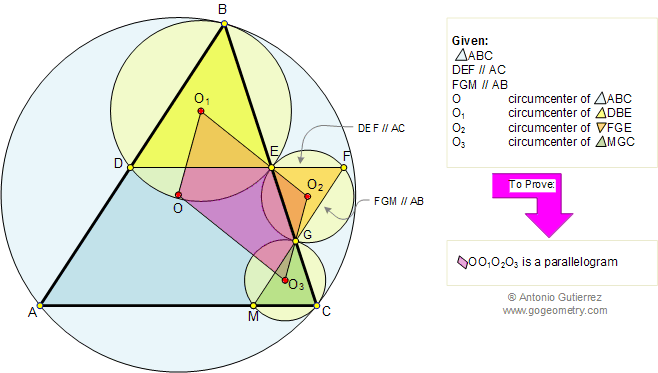# Problem 93. Similar Triangles, Circumcircles, Parallelogram. High School, College

 In the figure below, given a triangle ABC, line DEF parallel to AC and line FGM parallel to AB. If O, O1, O2, and O3, are the circumcenters of triangles ABC, DBE, FGE, and MGC respectively, prove that the quadrilateral OO1O2O3 is a parallelogram. View or post a solution.FACTS AND HINTS:

1. SIMILAR TRIANGLES:
Proposition:
Corresponding angles of similar triangles are congruent.

2. PROVING THAT LINES ARE PARALLEL:
Proposition:
Two lines are parallel if a pair of corresponding angles are congruent.
Proposition: Two lines are parallel if a pair of alternate interior angles are congruent.

3. See Proposed Problem 92

Home | Geometry | Search | Problems | Similarity | Circumcircle | Parallelograms | All Problems | 91-100 | Visual Index | Email | View or post a solution | by Antonio Gutierrez